##### Pre-Calculus For DummiesBecause you spend a ton of time in pre-calculus working with trigonometric functions, you need to understand ratios. One important ratio in right triangles is the cosine. The cosine of an angle, or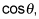is defined as the ratio of the adjacent leg to the hypotenuse, or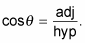Consider this example: A ladder leans against a building, creating an angle of 75 degrees with the ground. The base of the ladder is 3 feet away from the building. How long is the ladder? Did your heart just sink when you realized you’re dealing with a . . . word problem? No problem! Just follow these steps to solve; here, you’re looking for the length of the ladder:

1. Draw a picture so you can see a familiar shape.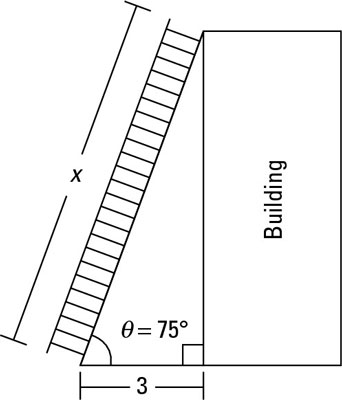One ladder plus one building equals one cosine problem.

The preceding figure represents the ladder leaning against the building.

The right angle goes between the building and the ground, because otherwise the building would be crooked and fall down. Because you know where the right angle is, you know that the hypotenuse is the ladder itself. The given angle is down on the ground, which means the opposite leg is the distance on the building from where the top of the ladder touches it to the ground. The third side, the adjacent leg, is the distance the bottom of the ladder rests from the building.

2. Set up a trigonometry equation, using the information from the picture.

You know that the adjacent side is 3 feet, and you’re looking for the length of the ladder, or the hypotenuse. Therefore, you have to use the cosine ratio, because it’s the ratio of the adjacent leg to the hypotenuse. You have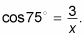The building has nothing to do with this problem right now, other than it’s what’s holding up the ladder.

Why do you use 75 degrees in the cosine function? Because you are told that the angle the ladder makes with the ground is 75 degrees. Thus you don’t have to use theta to represent an unknown angle.

3. Solve for the unknown variable.

Multiply the unknown x to both sides to get x cos 75 degrees = 3. Now divide both sides by cos 75 degrees to isolate x; you get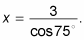The cos 75 degrees is just a number. When you plug it into your calculator, you get a decimal answer (make sure you set your calculator to degree mode before attempting to do this problem). This equation produces the (approximate) answer x = 11.6, which means the ladder is about 11.6 feet long.